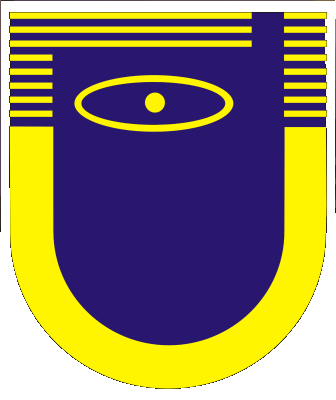Main Page
Subscription information

Current Issue
Tables of Contents
Author Index
Search

Authors
Referees

## Numerical investigation of electromagnetic effects and averaged metal melt flows generated by high frequency alternating magnetic field

I. L. Nikulin - A. V. Perminov

Perm National Research Politechnical University, Perm, Russia

Abstract
We describe in detail a mathematical model of averaged flows generated by an external high-frequency magnetic field in a molten metal. The model involves an eddy current magnetic field and a fluid dynamics problem. A system of equations for the eddy current magnetic field is given. This system allows to calculate harmonics of the eddy current magnetic field by standard numerical methods. A system of equations for metal flows in terms of the eddy field problem has been derived with the use of a multiple time scale method. The system was split into equations for averaged physical characteristics and for their pulsations. It is shown that the external alternating magnetic field generates time-independent volume forces and heat sources. Based on the results of the numerical simulations, the eddy current magnetic field distribution in molten metal and its influence on the averaged flows were analyzed. The averaged flow patterns are given. An application of this model is proposed. Figs 2, Refs 14.

Magnetohydrodynamics 52, No. 1/2, 135-144, 2016 [PDF, 0.61 Mb]

Copyright: Institute of Physics, University of Latvia
Electronic edition ISSN 1574-0579
Printed edition ISSN 0024-998X
DOI: http://doi.org/10.22364/mhd